Test: Logic Gates & Switching Circuits- 1

# Test: Logic Gates & Switching Circuits- 1

Test Description

## 10 Questions MCQ Test GATE Electrical Engineering (EE) 2023 Mock Test Series | Test: Logic Gates & Switching Circuits- 1

Test: Logic Gates & Switching Circuits- 1 for Electronics and Communication Engineering (ECE) 2023 is part of GATE Electrical Engineering (EE) 2023 Mock Test Series preparation. The Test: Logic Gates & Switching Circuits- 1 questions and answers have been prepared according to the Electronics and Communication Engineering (ECE) exam syllabus.The Test: Logic Gates & Switching Circuits- 1 MCQs are made for Electronics and Communication Engineering (ECE) 2023 Exam. Find important definitions, questions, notes, meanings, examples, exercises, MCQs and online tests for Test: Logic Gates & Switching Circuits- 1 below.
Solutions of Test: Logic Gates & Switching Circuits- 1 questions in English are available as part of our GATE Electrical Engineering (EE) 2023 Mock Test Series for Electronics and Communication Engineering (ECE) & Test: Logic Gates & Switching Circuits- 1 solutions in Hindi for GATE Electrical Engineering (EE) 2023 Mock Test Series course. Download more important topics, notes, lectures and mock test series for Electronics and Communication Engineering (ECE) Exam by signing up for free. Attempt Test: Logic Gates & Switching Circuits- 1 | 10 questions in 30 minutes | Mock test for Electronics and Communication Engineering (ECE) preparation | Free important questions MCQ to study GATE Electrical Engineering (EE) 2023 Mock Test Series for Electronics and Communication Engineering (ECE) Exam | Download free PDF with solutions
 1 Crore+ students have signed up on EduRev. Have you?
Test: Logic Gates & Switching Circuits- 1 - Question 1

### Match List-I (Logic gates) with List-ll (Symbols) and select the correct answer using the codes given below the lists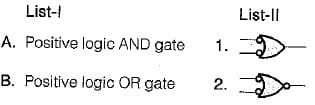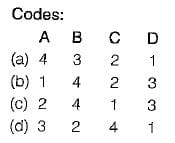Detailed Solution for Test: Logic Gates & Switching Circuits- 1 - Question 1
• Positive logic AND gate is equivalent to bubbled NOR gate.
• Positive logic OR gate is equivalent to bubbled NAND gate.
• Positive logic NAND gate is equivalent to bubbled OR gate.
• Positive logic NOR gate is equivalent to bubbled AND gate.
Test: Logic Gates & Switching Circuits- 1 - Question 2

### The minimum number of NAND gate required to implement the logic function given by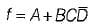is

Detailed Solution for Test: Logic Gates & Switching Circuits- 1 - Question 2can be implemented using 4 NAND gates as shown below: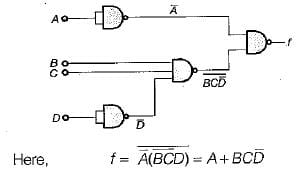Test: Logic Gates & Switching Circuits- 1 - Question 3

### Assertion (A): When Os and 1s are interchanged in the truth table, the positive logic AND gate becomes negative logic OR gate, and positive logic NAND gate becomes negative logic NOR gate. Reason (R): The simple method of converting the logic designation (i.e. from positive to negative logic or vice versa) is that all 0’s are replaced with 1s and all 1s with Os in the truth table.

Detailed Solution for Test: Logic Gates & Switching Circuits- 1 - Question 3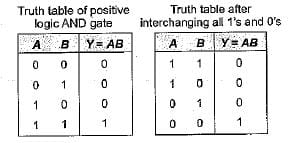Thus, we see that after interchanging all 1’s and 0’s of inputs in the truth table of positive logic AND gate, the resulting truth table obtained is the truth table of negative logic OR gate. Similarly, we can prove that if all 0’s and 1's inputs in the truth table of NAND gate are interchanged, then the gate becomes negative logic NOR gate. Hence, both assertion and reason are true and reason is the correct explanation of assertion.

Test: Logic Gates & Switching Circuits- 1 - Question 4

Match List-I (Logic gates) with List-ll (Applications) and select the correct answer using the codes given below the lists:

List-i
A. Exclusive OR gate
B. NOT gate
C. Exclusive NOR gate
D. NOR gate

List-ll
1. As a controlled inverter
2. As an universal gate
3. Complementation
4. Even-odd parity check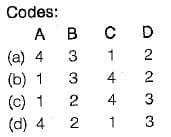Detailed Solution for Test: Logic Gates & Switching Circuits- 1 - Question 4
• EX-OR gate can be used as a controlled inverter as shown below: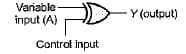For control input = 1 ,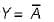For control input = 0, Y = A

• NOT gate is basically used as an inverter or for complementation purposes.
• EX-NOR gate can be used as even-odd parity checker because the output of EX- NOR gate is 1 if the number of 1’s in its inputs is even; if the number of 1’s is odd, the output is 0.
• NOR gate is an universal gate.
Test: Logic Gates & Switching Circuits- 1 - Question 5

Assertion (A): The EX-NOR gate can be used as a "one-bit comparator.

Reason (R): The output of the EX-NOR gate is high if the number of high inputs to it is even while the output is low if the number of high inputs to it is odd.

Detailed Solution for Test: Logic Gates & Switching Circuits- 1 - Question 5

An important property of the EX-NOR gate is that it can be used for bit comparison. The output of an EX-NOR gate is 1 (high) if both the inputs are similar, i.e. both are 0 or 1 (not necessarily even no. of high inputs); otherwise its output is 0 or low (not necessarily odd number of high inputs). Hence, EX-NOR gate can be used as a “one-bit comparator” . Thus, assertion is a correct statement. Reason is also a true statement but not a correct explanation of assertion.

Test: Logic Gates & Switching Circuits- 1 - Question 6

Consider the following logic circuit: What is the required input condition (A, B, C) to make the output X = 1, for the above logic circuit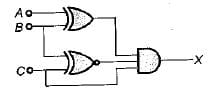Detailed Solution for Test: Logic Gates & Switching Circuits- 1 - Question 6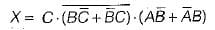Here, (0, 1, 1) gives the output X = 1

Test: Logic Gates & Switching Circuits- 1 - Question 7

Match List-I (Logic expression) with List-ll (Equivalent expression) and select the correct answer using the codes given below the lists: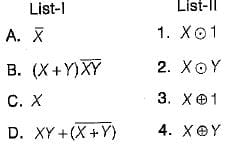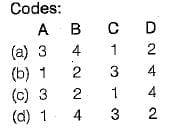Detailed Solution for Test: Logic Gates & Switching Circuits- 1 - Question 7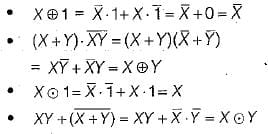Test: Logic Gates & Switching Circuits- 1 - Question 8

The code where all successive numbers differ from their preceding number by single bit is __________

Detailed Solution for Test: Logic Gates & Switching Circuits- 1 - Question 8

The code where all successive numbers differ from their preceding number by single bit is gray code. It is an unweighted code. The most important characteristic of this code is that only a single bit change occurs when going from one code number to next. BCD Code is one in which decimal digits are represented by a group of 4-bits each, whereas, in Excess-3 Code, the decimal numbers are incremented by 3 and then written in their BCD format.

Test: Logic Gates & Switching Circuits- 1 - Question 9

What is a Latch?

Detailed Solution for Test: Logic Gates & Switching Circuits- 1 - Question 9

It is a D-type of flip-flop and stores one bit of data.

Test: Logic Gates & Switching Circuits- 1 - Question 10

Which of the following logic gate follow both commutative and associate law?

Detailed Solution for Test: Logic Gates & Switching Circuits- 1 - Question 10

AND, OR, NOT are three basic gates which follow both commutative and associate law. However, the universal gates NAND and NOR don't follow associate law.

## GATE Electrical Engineering (EE) 2023 Mock Test Series

22 docs|274 tests
 Use Code STAYHOME200 and get INR 200 additional OFF Use Coupon Code
Information about Test: Logic Gates & Switching Circuits- 1 Page
In this test you can find the Exam questions for Test: Logic Gates & Switching Circuits- 1 solved & explained in the simplest way possible. Besides giving Questions and answers for Test: Logic Gates & Switching Circuits- 1, EduRev gives you an ample number of Online tests for practice

## GATE Electrical Engineering (EE) 2023 Mock Test Series

22 docs|274 tests